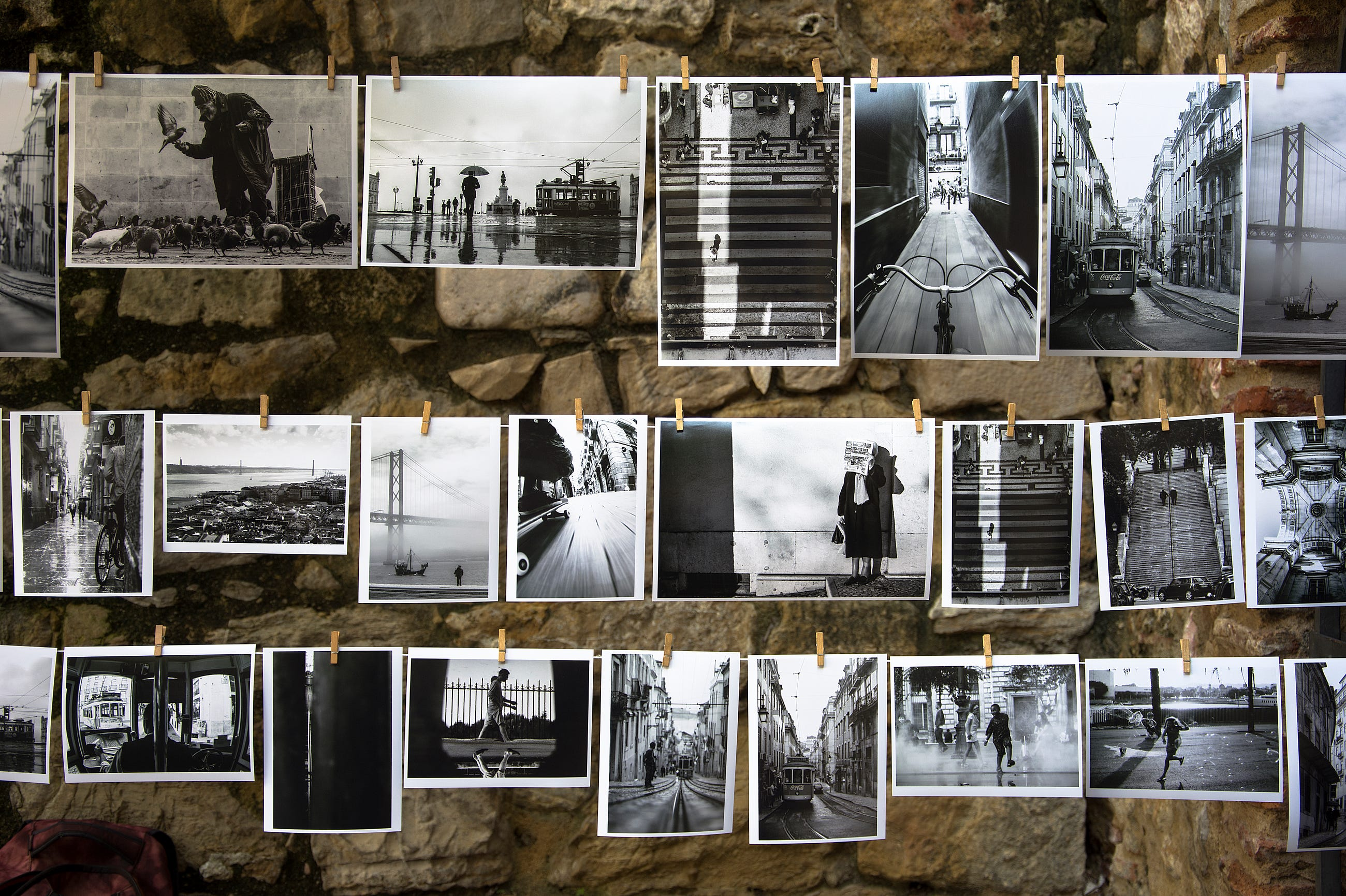Photo by Brigitta Schneiter

# GAN — CGAN & InfoGAN (using labels to improve GAN)

In discriminative models, like classification, we handcraft features to make models performing better. This practice is not needed if the model has enough capacity and it knows how to learn those features itself. In GAN, training models are non-trivial. It can take extra help from the labels to make the model performing better.

In CGAN (Conditional GAN), labels act as an extension to the latent space z to generate and discriminate images better. The top figure below is the regular GAN and the bottom adds labels to the generator and the discriminator to train both networks better.

The whole mechanism is still not fully understood. These labels may give a significant head start to GAN for what to look for. Another possibility is that our visual system is biased and more sensitive to these labels. Hence, the generated images are perceived to be better. Being part of a GAN series, this article studies how to improve the GAN performance with labels.

### CGAN (Conditional GAN)

In GAN, there is no control over modes of the data to be generated. The conditional GAN changes that by adding the label y as an additional parameter to the generator and hopes that the corresponding images are generated. We also add the labels to the discriminator input to distinguish real images better.

In MNIST, we sample the label y from a uniform distribution to generate a number from 0 to 9. We encode this value into a 1-hot vector. For example, the value 3 will be encoded as (0, 0, 0, 1, 0, 0, 0, 0, 0, 0). We feed the vector and the noise z to the generator to create an image that resembles “3”. For the discriminator, we add the supposed label as a one-hot vector to its input.

The cost function for CGAN is the same as GAN.

D(x|y) and G(z|y) demonstrates we are discriminating and generating an image given a label y. (It is the same as D(x, y) and G(z, y) in other diagrams.) Here is the data flow for CGAN.

In CGAN, we can expand the mechanism to include other labels that the training dataset may provide. For example, if the stroke size of the digits is known, we can sample it from a normal distribution and add that in generating images.

InfoGAN

The label y in CGAN are provided in the dataset. Alternatively, we can use our discriminator to extract all these latent features. In the example below, we sample a single feature c from a uniform distribution and convert it to a 1-hot vector. Then the generator uses this vector and z to generate an image.

When we feed images into the discriminator, it outputs D(x) and an additional output: a probability distribution Q(c|x) (the probability distribution for c given the image x.) For example, given a generated image resemble the digit “3”, Q may be estimated as (0.1, 0, 0, 0.8, …) meaning 0.1 chance that the image is a digit “0” and 0.8 chance that it is a “3”.

We subtract the regular GAN cost function with an extra term I(x; y) to form our new cost function.

I (mutual information) measures how much we know x if we know y. I(c;x) equals to 0 if the image x and the estimated c is completely irrelevant. Otherwise, if the discriminator can correctly predict c, I will be high and reduce the InfoGAN cost.

Without proof, the mutual information I can be estimated using entropy. We use Q(c|x) and P(C) to establish a lower bound for I.

where H stands for entropy. When the model performs, I will converge to its lower bound. The concept of mutual information may take time to settle in. However, it is pretty simple in coding.

``# If the image is a "3"# p_c = P(c) = [0, 0, 0, 1.0, 0, 0, 0, 0, 0, 0]``
`# The first term for I = - ∑ P(c) * log Q(c|x)`cross_H_p_q = tf.reduce_mean(                -tf.reduce_sum(p_c * tf.log(Q_c_given_x + 1e-8), 1))``
``# The entropy of c: H(c) = - `∑ `P(c) * log(P(c)) H_c = tf.reduce_mean(-tf.reduce_sum(p_c * tf.log(p_c + 1e-8), 1))``

### Further readings

If you want to learn more about GANs:

Or you want to know why it is so hard to train GANs:

### Credits & Reference

Conditional GAN paper

InfoGAN paper